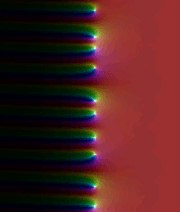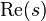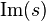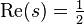# Riemann hypothesis facts for kids

Kids Encyclopedia FactsThe Riemann zeta function, in the complex plane. The real part$\operatorname{Re}(s)$ of the number is drawn horizontally, the imaginary part$\operatorname{Im}(s)$ vertically. White dots show the zeros where$\operatorname{Re}(s)=\tfrac{1}{2}$. Click to get a full view.

The Riemann hypothesis is a mathematical conjecture. Many people think that finding a proof of the hypothesis is one of the hardest and most important unsolved problems of pure mathematics.

The hypothesis is named after Bernhard Riemann. It is about a special function, the Riemann zeta function. This function inputs and outputs complex number values. The inputs that give the output zero are called zeros of the zeta function. Many zeros have been found. The "obvious" ones to find are the negative even integers. This follows from Riemann's functional equation. More have been computed and have real part 1/2. The hypothesis states all the undiscovered zeros must have real part 1/2.

The functional equation also says all zeros (except the "obvious" ones) must be in the critical strip: real part is between 0 and 1. The Riemann hypothesis says more: they are on the line given, in the image on the right (the white dots). If the hypothesis is false, this would mean that there are white dots which are not on the line given.

If proven correct, this would allow mathematicians to better describe how the prime numbers are placed among whole numbers. The Riemann hypothesis is so important, and so difficult to prove, that the Clay Mathematics Institute has offered \$1,000,000 to the first person to prove it.

## Images for kidsRiemann hypothesis Facts for Kids. Kiddle Encyclopedia.